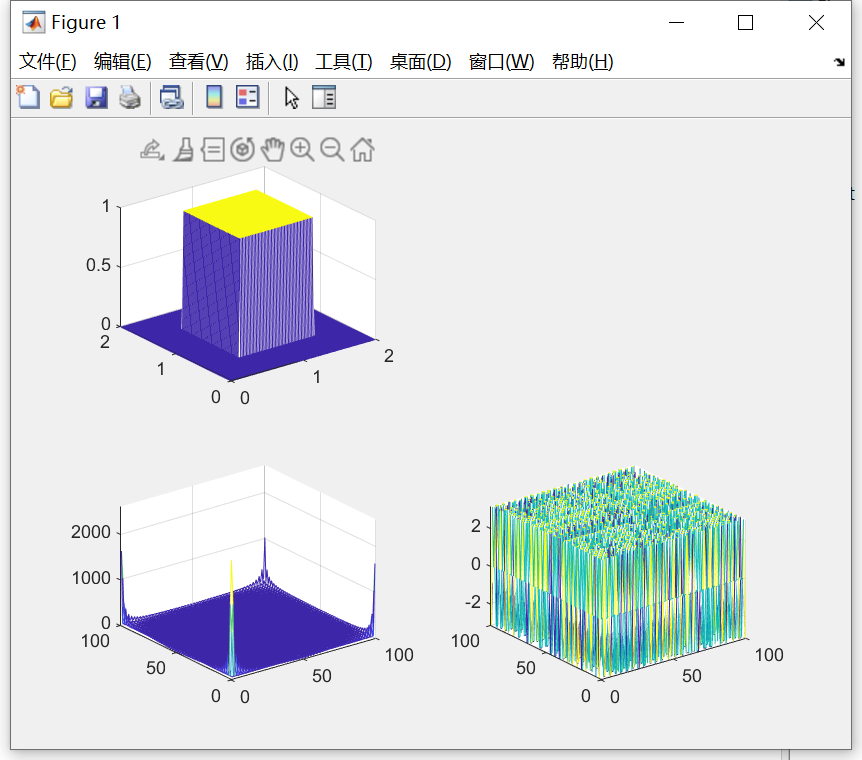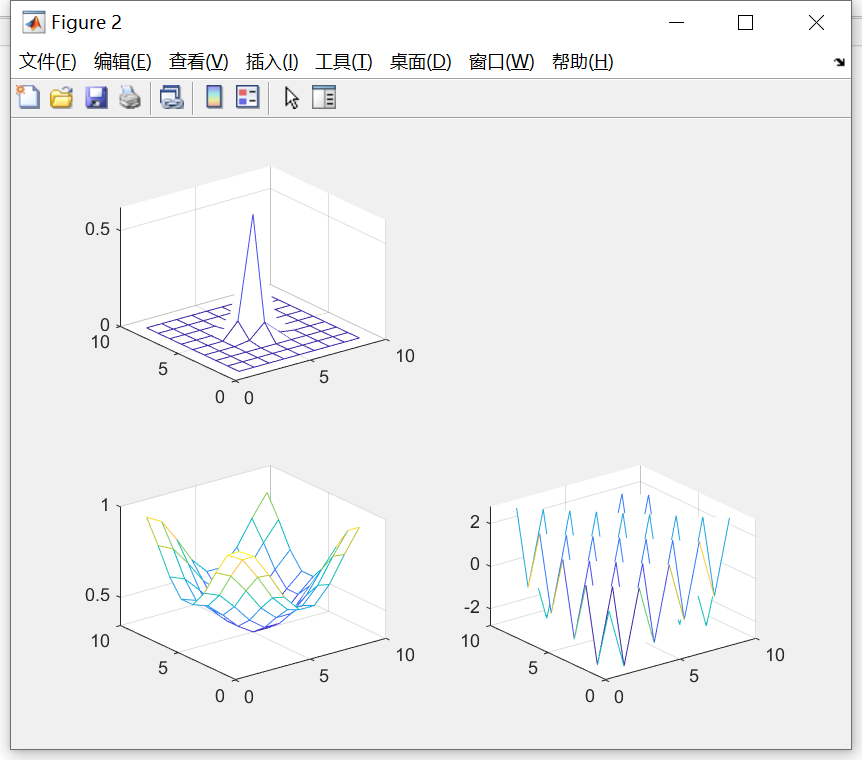• 前言
• 一、box函数
• 二、gaussian函数
• 注意

# 一、box函数

Fs = 50;

T = 1/Fs;

L = 100;

x = (0:L-1)*T;

y = (0:L-1)*T;

[X,Y]=meshgrid(x,y);

Z = 0.5<=X & X<=1.5 & 0.5<=Y & Y<=1.5;

Z_fft = fft2(Z);

figure;

subplot(2,2,1),mesh(X,Y,Z), grid on;

subplot(2,2,3),mesh(abs(Z_fft)), grid on;

subplot(2,2,4),mesh(angle(Z_fft)), grid on;# 二、gaussian函数

fspecial命令生成一个大小3*3，sigma为0.5的高斯方程


H = fspecial('gaussian',3*3,0.5);

Y = fft2(H);

AB=abs(Y);           % 计算频域序列 Y 的幅值
Pha=angle(Y);       % 计算频域序列 Y 的相角 (弧度制)

figure;

subplot(2,2,1),mesh(H), grid on;

subplot(2,2,3),mesh(AB), grid on;

subplot(2,2,4),mesh(Pha), grid on;# 注意

fft和fft2的区别：

fft是一维的傅里叶变换，是将时域信号转换为频域信号的；
fft2是二维的傅里叶变换，一般用来处理图像信息。